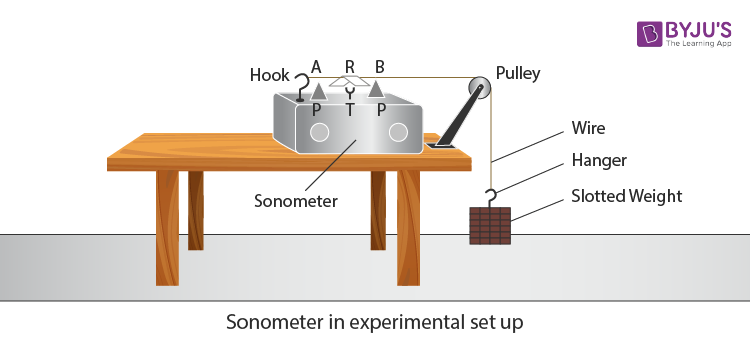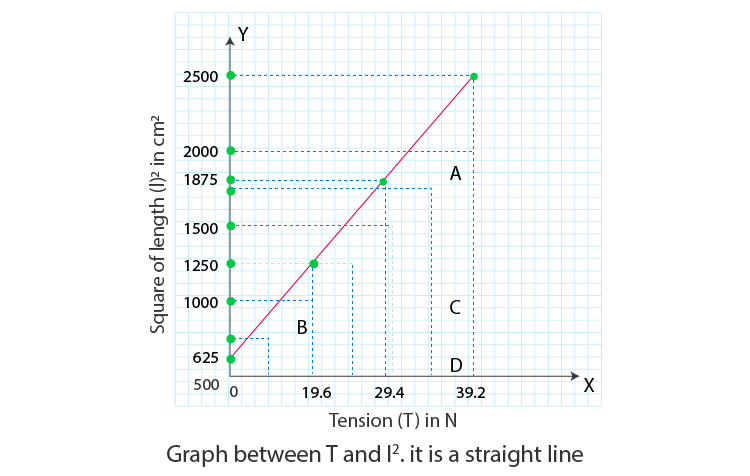# To Study The Relation Between The Length Of A Given Wire And Tension For Constant Frequency Using Sonometer

## Aim

To study the relation between the length of a given wire and tension for constant frequency using a sonometer.

## Materials Required

1. A sonometer
2. A set of eight tuning forks
3. ½ kg hanger
4. Seven ½ kg slotted weights
6. Paper rider
7. Metre-scale
8. Screw gauge

## Theory

The frequency of the string and the tuning fork will be the same if they vibrate at the same resonance. For a string with length l, diameter D, material density ρ and tension T, then the frequency v is given as:

 $$\begin{array}{l}v=\frac{1}{lD}\sqrt{\frac{T}{\pi \rho }}\end{array}$$

From above equation, vl = constant

A graph between v and 1/l will be a straight line and the graph between v and l will be a hyperbola. A graph between T and l2 will be a straight line as T ∝ l2.

## Diagram## Procedure

1. Place the sonometer with a load of 4 kg on the table as shown in the above diagram.
2. Frictionless pulley should be used for the experiment.
3. The hanger should carry a maximum weight that is suitable for it.
4. To have a maximum length of the wire, move the wooden bridges accordingly.
5. From the set of tuning fork, select 256 Hz fork and strike it against the rubber pad to make it vibrate. After striking the fork, bring it near your ear.
6. Leave the wire AB to vibrate by plucking it from the middle.
7. To determine the lowest frequency, the sound produced from tuning fork and wire should be compared.Note: Sound with low pitch will have less frequency.
8. Reduce the distance between the wooden bridges as the frequency from the wire is less. Check for the frequencies again.
9. The length of the wire AB should be reduced continuously until the frequency of the wire becomes equal to the frequency of the tuning fork.
10. Place a V shaped paper rider R in the middle of the wire. Strike the tuning fork against the rubber pad and touch the lower end of the handle with the sonometer board. The wire AB will start to vibrate and the rider R falls off the wire AB.
11. As the wooden bridges are moved closer the length of the wire AB reduces. Record the length of wire AB in the table under the “length decreasing” column.
12. Now bring the wooden bridges closer and adjust the length of the wire AB by increasing it till the rider R falls off.
13. Record the increase in the length of the wire under “length increasing” in the table.
14. Remove the slotted weights one by one and reduce the loads to 3.5 kg, 3 kg, etc till it reaches 1 kg. Repeat the above steps with the same fork.
15. Record the observations in a tabular form.

## Observations

Constant frequency of tuning fork, v = 256 Hz

Table for tension (load) and length:

 Sl. no Load M (kg) Tension Mg T(N) Resonant length of wire l2 (cm2) l2/T (cm2N-1) Length increasing l1 (cm) Length decreasing l2 (cm) Mean = ( l1+ l2)/2 l(cm) 1. 2. 3. 4. 5. 6. 7.

## Calculations

1. Find mean length l and write it in “mean length” column 4c.
2. Find l2 and write in l2 column 5.
3. Find l2/T and write in l2/T column 6.
4. Plot the graph between T and l2, taking T along the x-axis and l2 along the y-axis. The graph will be a straight line.## Result

1. From column 6, we found that l2/T = constant.
2. From the graph, it is concluded that T ∝ l2 for constant frequency v and it corresponds to the formula:
$$\begin{array}{l}v=\frac{1}{lD}\sqrt{\frac{T}{\pi \rho }}\end{array}$$

This verifies the law of tension of transverse vibrations of strings.

## Precautions

1. Frictionless pulleys should be used.
2. The wires should be of uniform cross-section and kinkless.
3. The maximum load on the wire should not exceed the elastic limit.
4. Soft rubber pad should be used to strike the tuning forks.
5. For calculations, the mean of length decreasing and increasing should be used.
6. While transferring the vibrations, the lower end of the handle should be touched gently to the sonometer board.
7. The load should include hanger weight too.
8. After the experiment, the load should be removed.

## Sources of Error

1. Frictionless pulley might not be used.
2. The rigid and uniform cross-sectional wire might not be used.
3. Correct weights are not used.
4. Sharpe wooden bridges might not be used.

## Viva Questions

Q1. What is the law of length?

Ans: Mathematical form of the law of length when T and m are constant is:

 $$\begin{array}{l}v\propto \frac{1}{l}\end{array}$$

Q2. What is the law of tension?

Ans: Mathematical form of the law of tension when l and m are constant is:

 $$\begin{array}{l}v\propto\sqrt{T}\end{array}$$

Q3. What is the law of mass?

Ans: Mathematical form of the law of mass when l and T are constant is:

 $$\begin{array}{l}v\propto\frac{1}{\sqrt{m}}\end{array}$$

Q4. What is pitch of sound and on what factor does it depend?

Ans: Pitch of sound is defined as the sensation of sound when it is either shrill or grave. It depends upon the frequency of sound.

Q5. What is a sonometer?

Ans: Sonometer is a hollow rectangular wooden box.